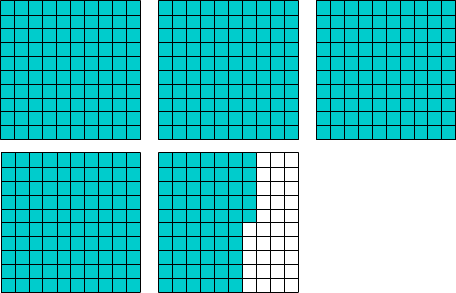# Decimal Illustration

Rate 0 stars Common Core
Quiz size:
Message preview:
Someone you know has shared Decimal Illustration quiz with you:

To play this quiz, click on the link below:

https://www.turtlediary.com/quiz/decimal-illustration.html

Hope you have a good experience with this site and recommend to your friends too.

Login to rate activities and track progress.
Login to rate activities and track progress.

Consider the model.Let's find the decimal number illustrated by this model.

There are four full grids. So, the whole number part is 4.

In the last grid, there are 100 squares out of which 65 are colored. So, the decimal part is sixty-five hundredths.

So, the part shaded in the model is four and sixty-five hundredths.

Now, let's write four and sixty-five hundredths as a decimal.

 Ones . Tenths Hundredths 4 . 6 5

Four and sixty-five hundredths is written as the decimal 4.65.

So, the decimal number illustrated by the given model is 4.65.

ds

A B C D E F G H I J K L M N O P Q R S T U V W X Y Z